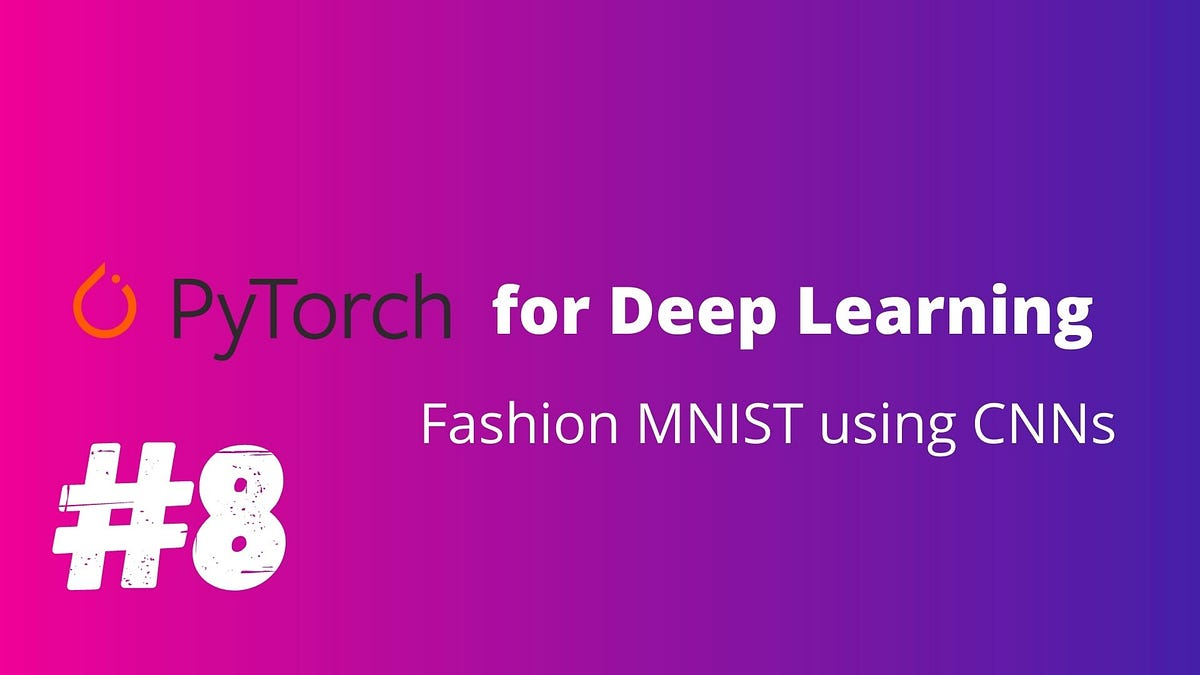# PyTorch For Deep Learning — Convolutional Neural Networks ( Fashion-MNIST )PyTorch For Deep Learning — Convolutional Neural Networks ( Fashion-MNIST ). This blog post is all about how to create a model to predict fashion mnist images and shows how to implement convolutional layers in the network.

## Fashion MNIST

Fashion Mnist is a Dataset created by Zolando Fashion Wear to replace the Original Mnist and at the same time increasing the difficulty.

This blog post is all about how to create a model to predict fashion mnist images and shows how to implement convolutional layers in the network

Let’s look at the code

## Convolutional Neural Networks

Basic fundamentals of CNN. CNN’s are a special type of ANN which accepts images as inputs. Below is the representation of a basic neuron of an ANN which takes as input X vector.

## Architecture and Training Of Convolutional Neural Networks (7 points):

This post provides the details of the architecture of Convolutional Neural Network (CNN), functions and training of each layer, ending with a summary of the training of CNN.

## Bayesian Neural Networks: 2 Fully Connected in TensorFlow and Pytorch

This chapter continues the series on Bayesian deep learning. In the chapter we’ll explore alternative solutions to conventional dense neural networks.

## Convolutional Neural Network: How is it different from the other networks?

Convolutional Neural Network: How is it different from the other networks? What’s so unique about CNNs and what does convolution really do? This is a math-free introduction to the wonders of CNNs.

## Convolutional Neural Networks(CNN’s) — A practical perspective

A dive into the main operations in a convolutional neural network plus classifying heartbeats using Mel-Spectrograms and transfer learning. n this blog, we will look into some concepts of CNN’s for image classification that are often missed or misunderstood by beginners.